# RD Sharma Solutions for Class 12 Maths Exercise 2.2 Chapter 2 Function

RD Sharma Solutions for Class 12 Maths Exercise 2.2 Chapter 2 Functions is provided here. For students who wish to excel in their exams and build their confidence level, practising RD Sharma Class 12 textbook is essential. Students can download the PDF of RD Sharma Solutions for Class 12 Exercise 2.2 of Chapter 2 Function from the links provided below. This exercise deals with the composition of functions. At BYJU’S, we have a set of experienced faculty in various fields designing answers for the exercise-wise problems of RD Sharma textbook. Understanding the concepts and clearing the doubts immediately during self-study is the main aim of providing exercise-wise solutions in PDF format.

## Download the PDF of RD Sharma Solutions for Class 12 Chapter 2 – Function Exercise 2.2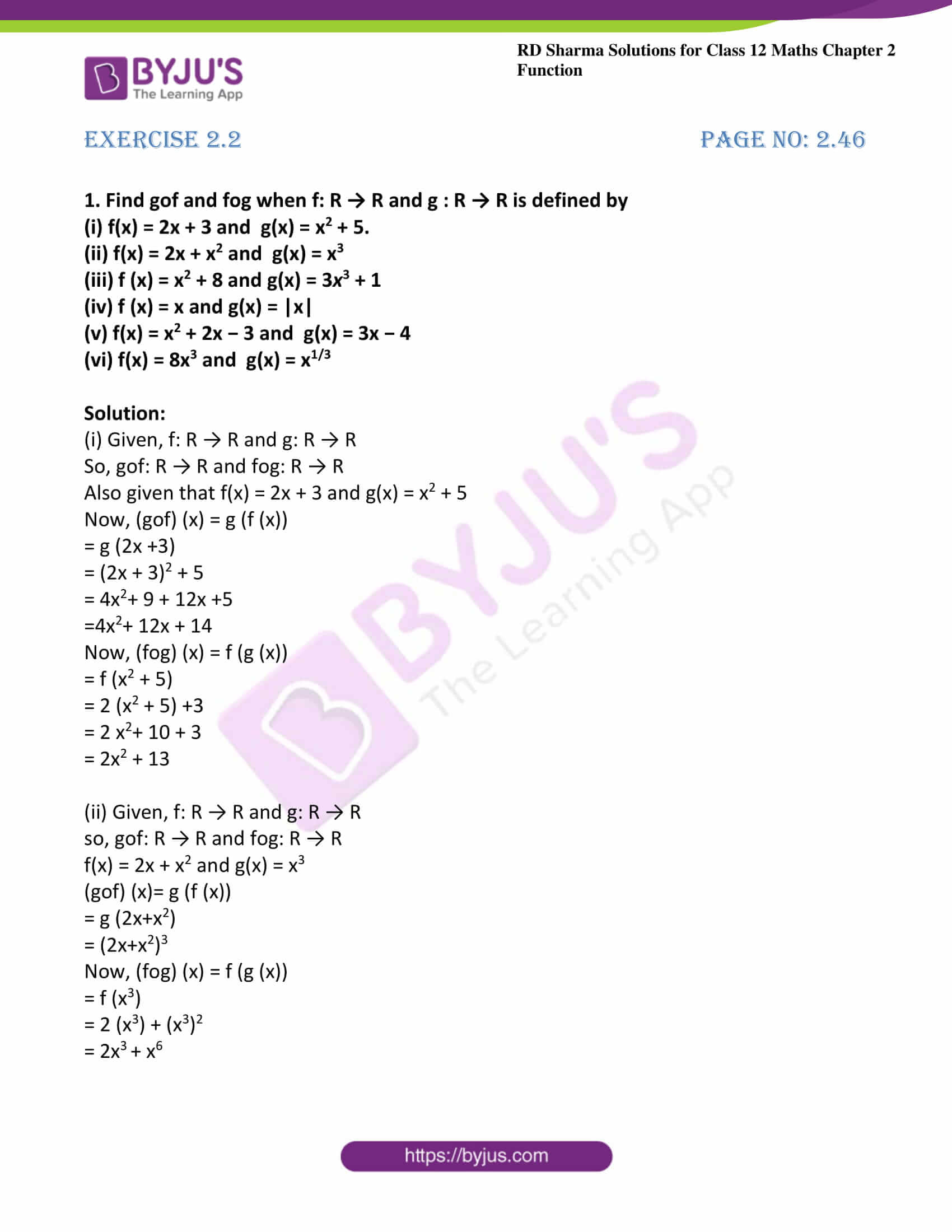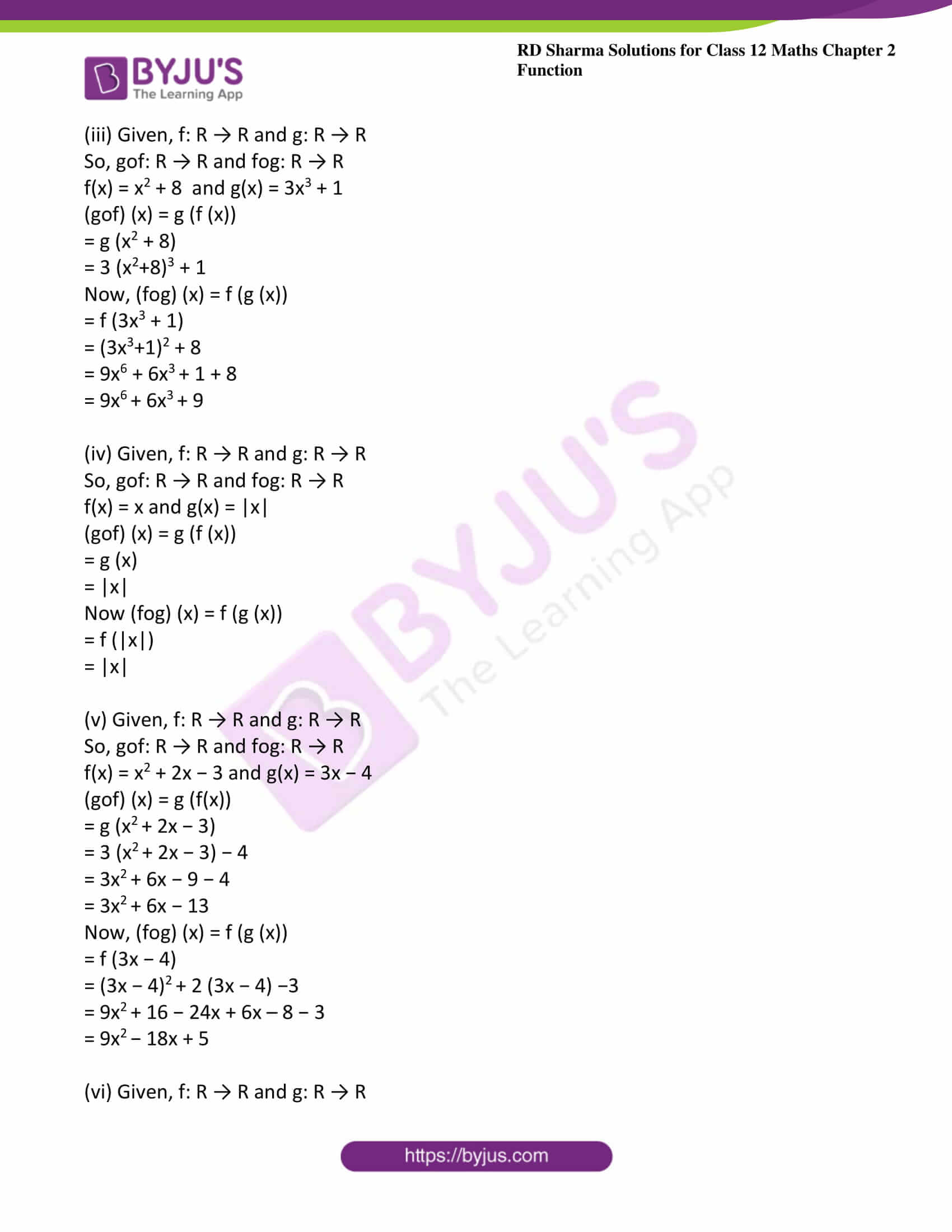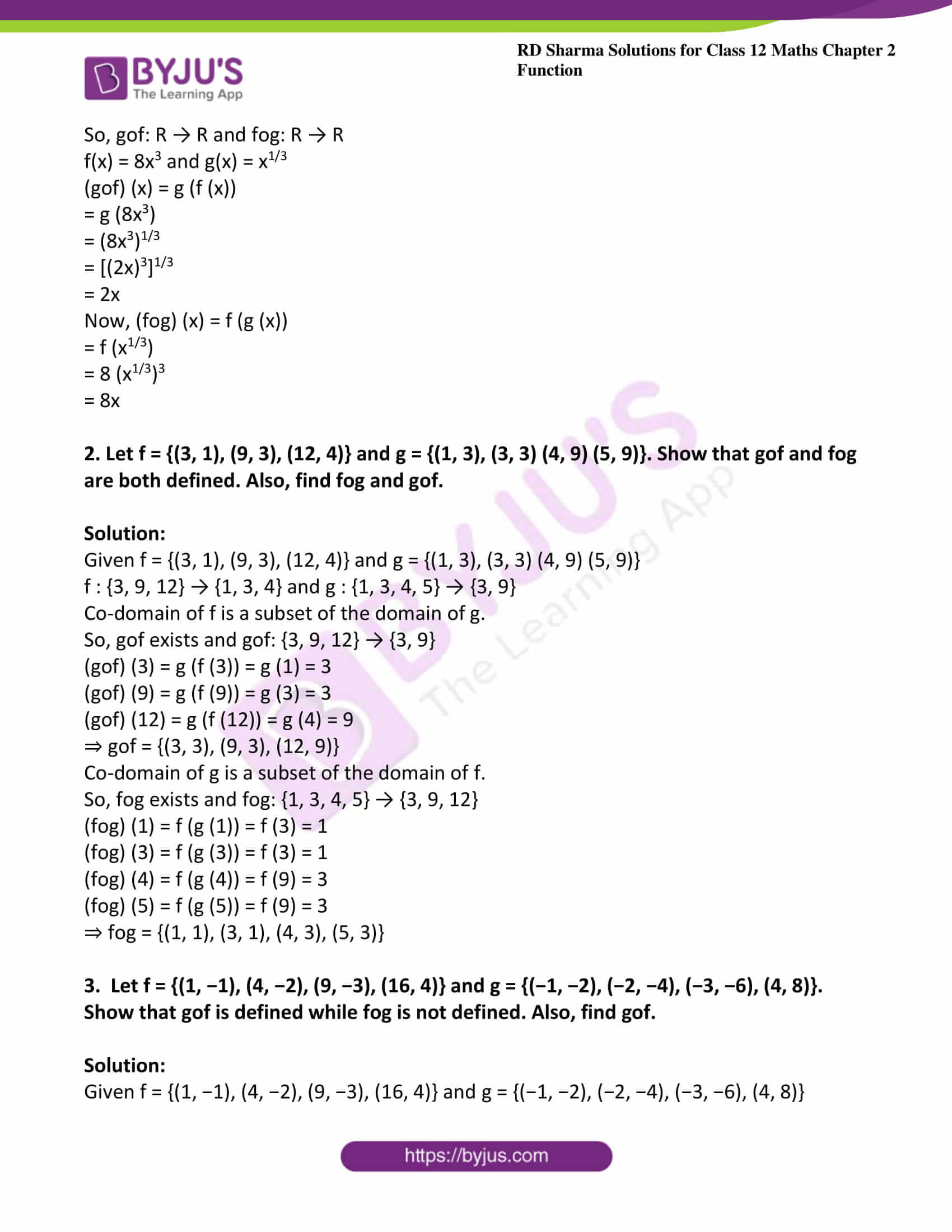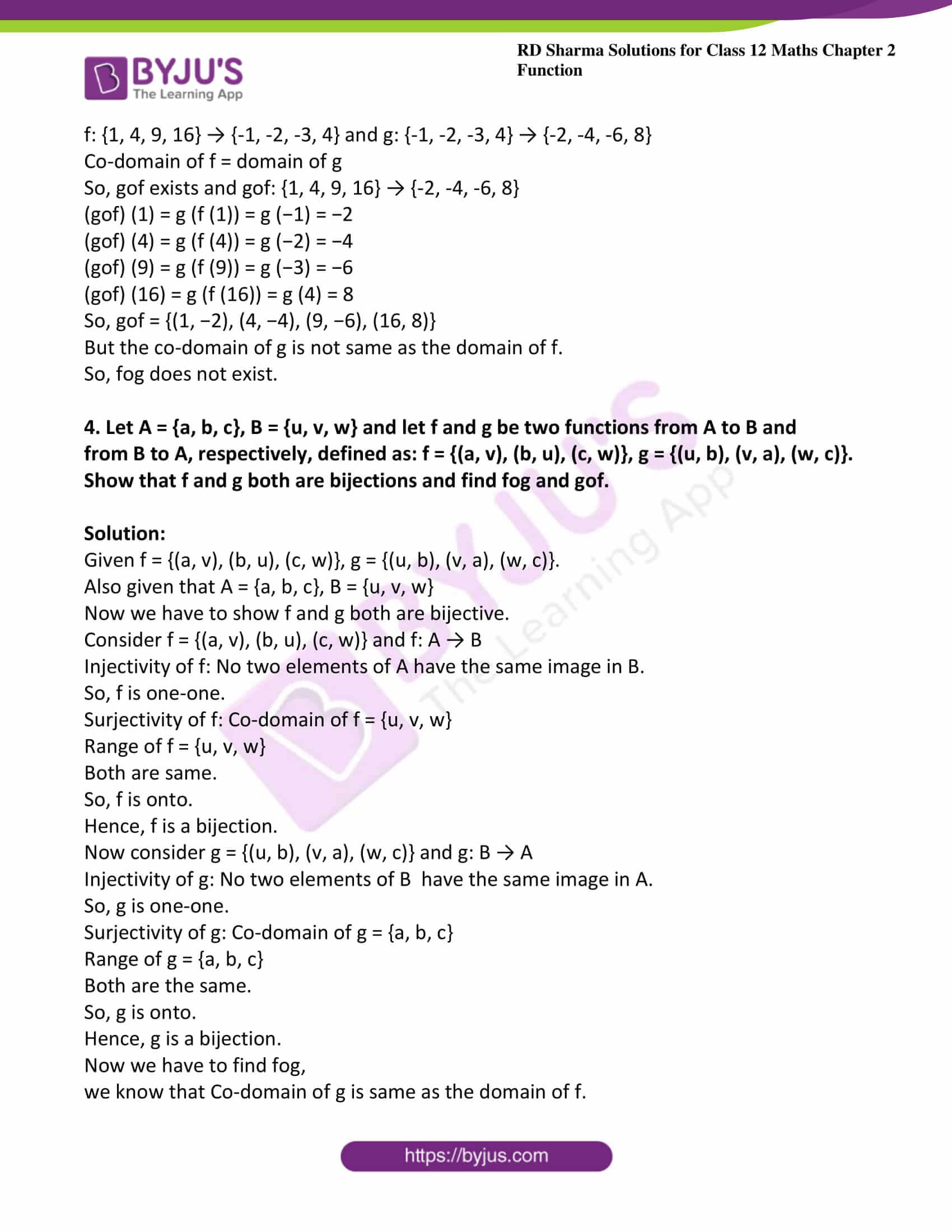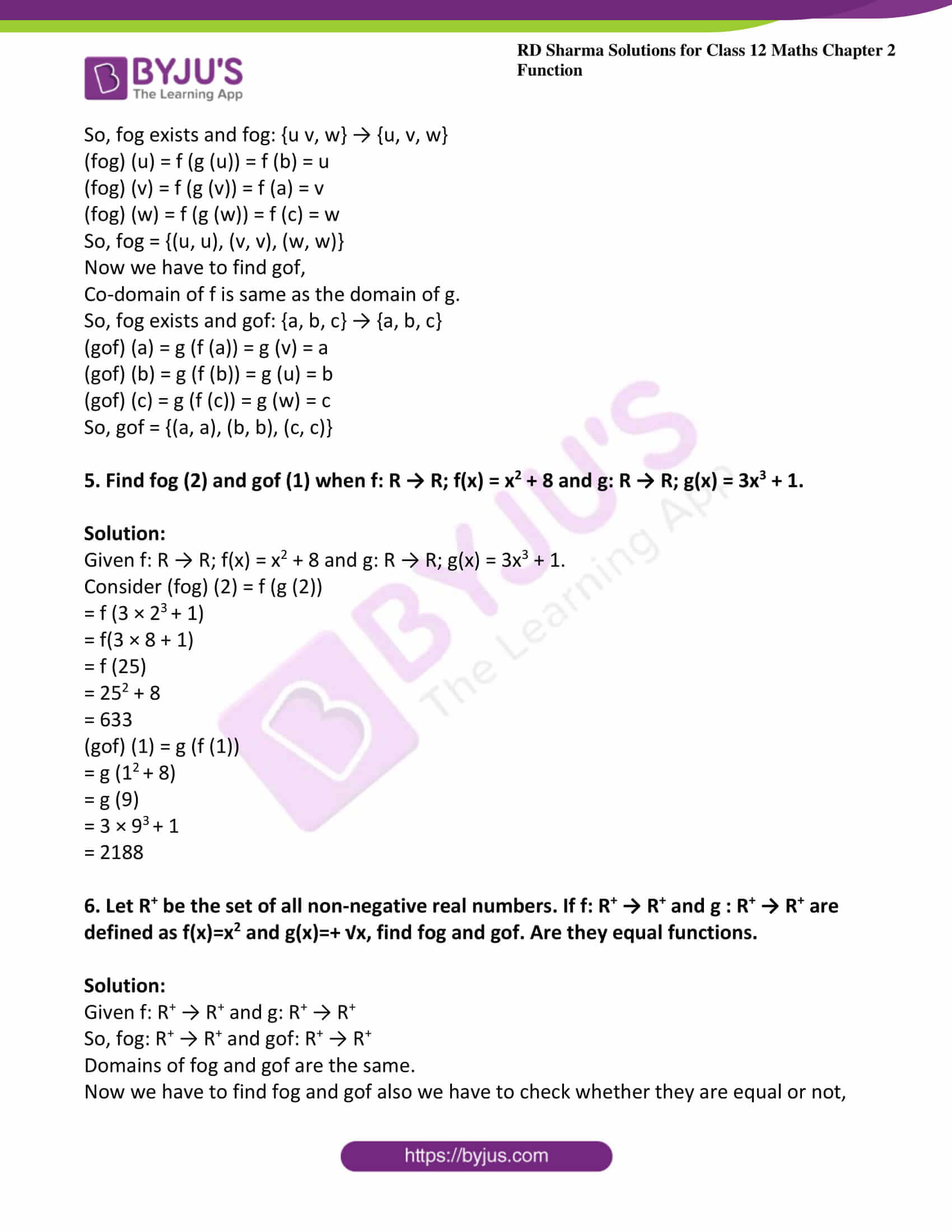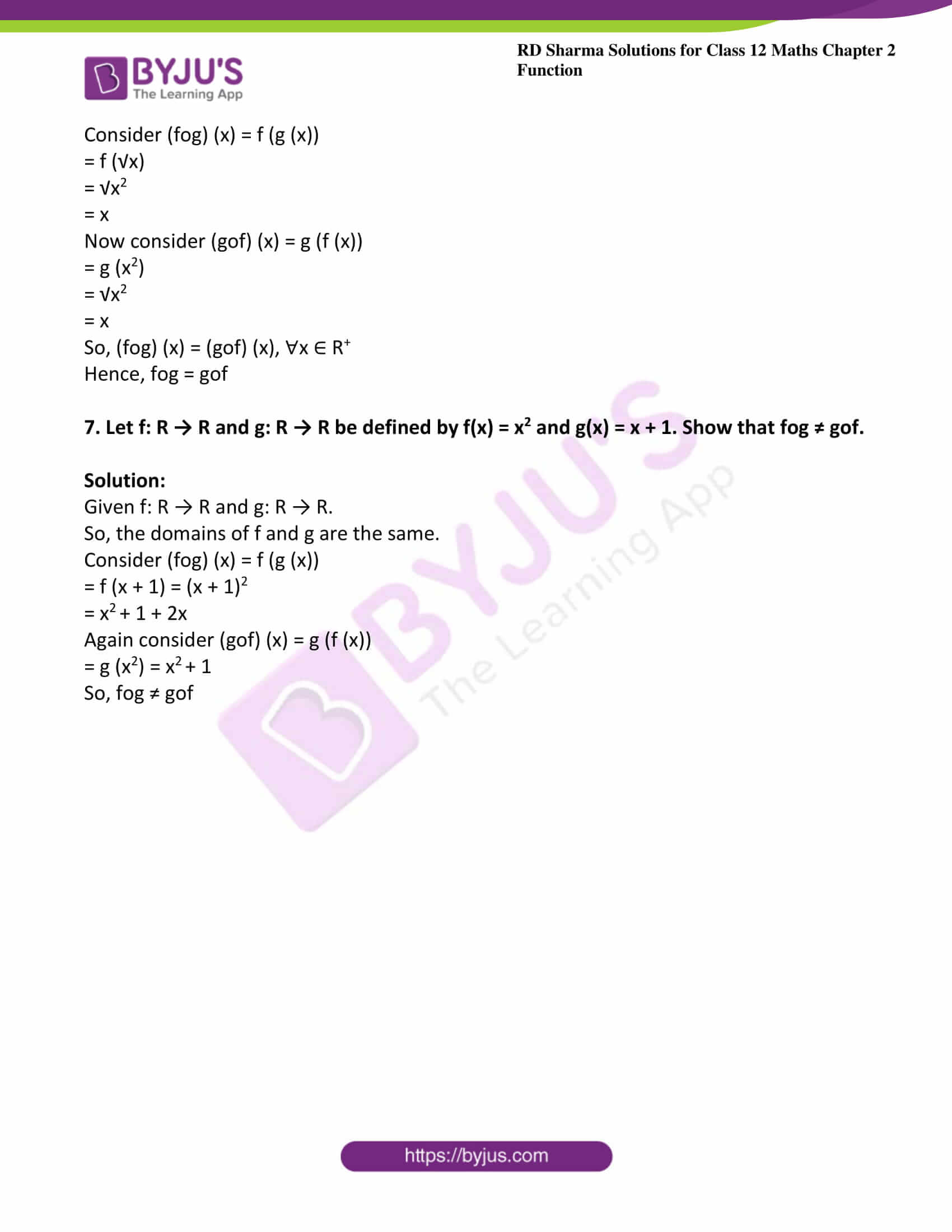### Access other exercises of RD Sharma Solutions For Class 12 Chapter 2 – Function

Exercise 2.1 Solutions

Exercise 2.3 Solutions

Exercise 2.4 Solutions

### Exercise 2.2 Page No: 2.46

1. Find gof and fog when f: R → R and g : R → R is defined by

(i) f(x) = 2x + 3 and  g(x) = x2 + 5.

(ii) f(x) = 2x + x2 and  g(x) = x3

(iii) f (x) = x2 + 8 and g(x) = 3x3 + 1

(iv) f (x) = x and g(x) = |x|

(v) f(x) = x2 + 2x − 3 and  g(x) = 3x − 4

(vi) f(x) = 8x3 and  g(x) = x1/3

Solution:

(i) Given, f: R → R and g: R → R

So, gof: R → R and fog: R → R

Also given that f(x) = 2x + 3 and g(x) = x2 + 5

Now, (gof) (x) = g (f (x))

= g (2x +3)

= (2x + 3)2 + 5

= 4x2+ 9 + 12x +5

=4x2+ 12x + 14

Now, (fog) (x) = f (g (x))

= f (x2 + 5)

= 2 (x2 + 5) +3

= 2 x2+ 10 + 3

= 2x2 + 13

(ii) Given, f: R → R and g: R → R

so, gof: R → R and fog: R → R

f(x) = 2x + x2 and g(x) = x3

(gof) (x)= g (f (x))

= g (2x+x2)

= (2x+x2)3

Now, (fog) (x) = f (g (x))

= f (x3)

= 2 (x3) + (x3)2

= 2x3 + x6

(iii) Given, f: R → R and g: R → R

So, gof: R → R and fog: R → R

f(x) = x2 + 8  and g(x) = 3x3 + 1

(gof) (x) = g (f (x))

= g (x2 + 8)

= 3 (x2+8)3 + 1

Now, (fog) (x) = f (g (x))

= f (3x3 + 1)

= (3x3+1)2 + 8

= 9x6 + 6x3 + 1 + 8

= 9x6 + 6x3 + 9

(iv) Given, f: R → R and g: R → R

So, gof: R → R and fog: R → R

f(x) = x and g(x) = |x|

(gof) (x) = g (f (x))

= g (x)

= |x|

Now (fog) (x) = f (g (x))

= f (|x|)

= |x|

(v) Given, f: R → R and g: R → R

So, gof: R → R and fog: R → R

f(x) = x2 + 2x − 3 and g(x) = 3x − 4

(gof) (x) = g (f(x))

= g (x2 + 2x − 3)

= 3 (x2 + 2x − 3) − 4

= 3x2 + 6x − 9 − 4

= 3x2 + 6x − 13

Now, (fog) (x) = f (g (x))

= f (3x − 4)

= (3x − 4)2 + 2 (3x − 4) −3

= 9x2 + 16 − 24x + 6x – 8 − 3

= 9x2 − 18x + 5

(vi) Given, f: R → R and g: R → R

So, gof: R → R and fog: R → R

f(x) = 8x3 and g(x) = x1/3

(gof) (x) = g (f (x))

= g (8x3)

= (8x3)1/3

= [(2x)3]1/3

= 2x

Now, (fog) (x) = f (g (x))

= f (x1/3)

= 8 (x1/3)3

= 8x

2. Let f = {(3, 1), (9, 3), (12, 4)} and g = {(1, 3), (3, 3) (4, 9) (5, 9)}. Show that gof and fog are both defined. Also, find fog and gof.

Solution:

Given f = {(3, 1), (9, 3), (12, 4)} and g = {(1, 3), (3, 3) (4, 9) (5, 9)}

f : {3, 9, 12} → {1, 3, 4} and g : {1, 3, 4, 5} → {3, 9}

Co-domain of f is a subset of the domain of g.

So, gof exists and gof: {3, 9, 12} → {3, 9}

(gof) (3) = g (f (3)) = g (1) = 3

(gof) (9) = g (f (9)) = g (3) = 3

(gof) (12) = g (f (12)) = g (4) = 9

⇒ gof = {(3, 3), (9, 3), (12, 9)}

Co-domain of g is a subset of the domain of f.

So, fog exists and fog: {1, 3, 4, 5} → {3, 9, 12}

(fog) (1) = f (g (1)) = f (3) = 1

(fog) (3) = f (g (3)) = f (3) = 1

(fog) (4) = f (g (4)) = f (9) = 3

(fog) (5) = f (g (5)) = f (9) = 3

⇒ fog = {(1, 1), (3, 1), (4, 3), (5, 3)}

3.  Let f = {(1, −1), (4, −2), (9, −3), (16, 4)} and g = {(−1, −2), (−2, −4), (−3, −6), (4, 8)}. Show that gof is defined while fog is not defined. Also, find gof.

Solution:

Given f = {(1, −1), (4, −2), (9, −3), (16, 4)} and g = {(−1, −2), (−2, −4), (−3, −6), (4, 8)}

f: {1, 4, 9, 16} → {-1, -2, -3, 4} and g: {-1, -2, -3, 4} → {-2, -4, -6, 8}

Co-domain of f = domain of g

So, gof exists and gof: {1, 4, 9, 16} → {-2, -4, -6, 8}

(gof) (1) = g (f (1)) = g (−1) = −2

(gof) (4) = g (f (4)) = g (−2) = −4

(gof) (9) = g (f (9)) = g (−3) = −6

(gof) (16) = g (f (16)) = g (4) = 8

So, gof = {(1, −2), (4, −4), (9, −6), (16, 8)}

But the co-domain of g is not the same as the domain of f.

So, fog does not exist.

4. Let A = {a, b, c}, B = {u, v, w} and let f and g be two functions from A to B and from B to A, respectively, defined as: f = {(a, v), (b, u), (c, w)}, g = {(u, b), (v, a), (w, c)}.
Show that f and g both are bijections and find fog and gof.

Solution:

Given f = {(a, v), (b, u), (c, w)}, g = {(u, b), (v, a), (w, c)}.

Also given that A = {a, b, c}, B = {u, v, w}

Now we have to show f and g both are bijective.

Consider f = {(a, v), (b, u), (c, w)} and f: A → B

Injectivity of f: No two elements of A have the same image in B.

So, f is one-one.

Surjectivity of f: Co-domain of f = {u, v, w}

Range of f = {u, v, w}

Both are the same.

So, f is onto.

Hence, f is a bijection.

Now consider g = {(u, b), (v, a), (w, c)} and g: B → A

Injectivity of g: No two elements of B  have the same image in A.

So, g is one-one.

Surjectivity of g: Co-domain of g = {a, b, c}

Range of g = {a, b, c}

Both are the same.

So, g is onto.

Hence, g is a bijection.

Now we have to find fog,

we know that the co-domain of g is the same as the domain of f.

So, fog exists and fog: {u v, w} → {u, v, w}

(fog) (u) = f (g (u)) = f (b) = u

(fog) (v) = f (g (v)) = f (a) = v

(fog) (w) = f (g (w)) = f (c) = w

So, fog = {(u, u), (v, v), (w, w)}

Now we have to find gof,

Co-domain of f is the same as the domain of g.

So, fog exists and gof: {a, b, c} → {a, b, c}

(gof) (a) = g (f (a)) = g (v) = a

(gof) (b) = g (f (b)) = g (u) = b

(gof) (c) = g (f (c)) = g (w) = c

So, gof = {(a, a), (b, b), (c, c)}

5. Find fog (2) and gof (1) when f: R → R; f(x) = x2 + 8 and g: R → R; g(x) = 3x3 + 1.

Solution:

Given f: R → R; f(x) = x2 + 8 and g: R → R; g(x) = 3x3 + 1.

Consider (fog) (2) = f (g (2))

= f (3 × 23 + 1)

= f(3 × 8 + 1)

= f (25)

= 252 + 8

= 633

(gof) (1) = g (f (1))

= g (12 + 8)

= g (9)

= 3 × 93 + 1

= 2188

6. Let R+ be the set of all non-negative real numbers. If f: R+ → R+ and g : R+ → R+ are defined as f(x)=x2 and g(x)=+ √x, find fog and gof. Are they equal functions.

Solution:

Given f: R+ → R+ and g: R+ → R+

So, fog: R+ → R+ and gof: R+ → R+

Domains of fog and gof are the same.

Now we have to find fog and gof also we have to check whether they are equal or not,

Consider (fog) (x) = f (g (x))

= f (√x)

= √x2

= x

Now consider (gof) (x) = g (f (x))

= g (x2)

= √x2

= x

So, (fog) (x) = (gof) (x), ∀x ∈ R+

Hence, fog = gof

7. Let f: R → R and g: R → R be defined by f(x) = x2 and g(x) = x + 1. Show that fog ≠ gof.

Solution:

Given f: R → R and g: R → R.

So, the domains of f and g are the same.

Consider (fog) (x) = f (g (x))

= f (x + 1) = (x + 1)2

= x2 + 1 + 2x

Again consider (gof) (x) = g (f (x))

= g (x2) = x2 + 1

So, fog ≠ gof